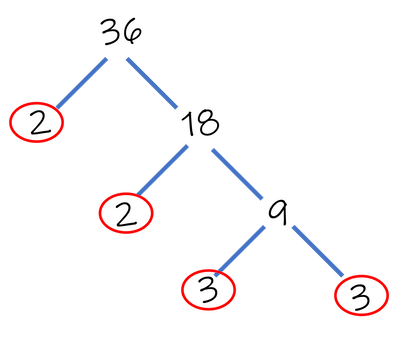// StartMathJax Script window.MathJax = {loader: {load: [ 'input/asciimath', 'ui/lazy', 'output/chtml', 'ui/menu']} }; (function() { var script = document.createElement('script'); script.src = "https://cdn.jsdelivr.net/npm/mathjax@3/es5/startup.js"; script.async = true; document.head.appendChild(script); })(); ---------- (Different files) ---------- // UpdateTypeset Script config = { attributes: true, childList: true, subtree: true }; // Callback function to execute when mutations are observed callback = (mutationList, observer) => { for (mutation of mutationList) { if (mutation.type === 'childList') { console.log('A child node has been added or removed.'); MathJax.typeset(); } else if (mutation.type === 'attributes') { console.log(The \${mutation.attributeName} attribute was modified.); } } }; // Create an observer instance linked to the callback function observer = new MutationObserver(callback); document.onreadystatechange = () => { if (document.readyState === 'complete') { console.log("Loaded fully according to readyState") targetNode = document.getElementById('content-wrapper') console.log(targetNode) // Start observing the target node for configured mutations observer.observe(targetNode, config); } }
top of page
Search

# Prime Factorisation

Simply Maths

Prime factorisation is a way of expressing a number as a product of its prime factors. To do prime factorisation, you need to find the prime numbers that, when multiplied together, give you the original number. Here's a step-by-step guide on how to do prime factorisation:

Let's use the number 36 as an example.

Find the Smallest Prime Divisor

Start with the smallest prime number, which is 2. Check if your number is divisible by 2. If it is, divide the number by 2 and write down the factor. If it's not divisible by 2, move on to the next prime number (3), and so on.

In our example, 36 is divisible by 2, so we divide it by 2:

36 ÷ 2 = 18

So, we have the factor 2.

Continue Dividing by Prime Factors

Repeat the process with the quotient from the previous step (18). Find the smallest prime factor that divides the quotient and continue dividing until you reach a prime number.

18 is divisible by 2 again:

18 ÷ 2 = 9

So, we have another factor of 2.

Now, continue with the quotient (9):

9 is not divisible by 2 but is divisible by 3:

9 ÷ 3 = 3

So, we have another factor of 3.

Continue with the quotient (3):

3 is already a prime number, so we don't need to divide further.

Write Down the Prime Factors

Now that you have completely factored the number, write down all the prime factors you found. In this example, the prime factorisation of 36 in expanded form is:

2 × 2 × 3 × 3

In exponent form: 2² × 3²

Factor trees help to make the process and result clearer (notice I've circled the prime factors):Let's do another example:

Example 2: Prime Factorisation of 48

2. Divide by the smallest prime factor (2): 48 ÷ 2 = 24.

3. Divide again by 2: 24 ÷ 2 = 12.

4. Divide by 2 again: 12 ÷ 2 = 6.

5. Divide by the next smallest prime (3): 6 ÷ 3 = 2.

6. Finish since 2 is prime.

As a factor tree:Now, write down the prime factorisation:

In expanded form: 2 × 2 × 2 × 2 × 3

In exponent form: 2⁴ × 3